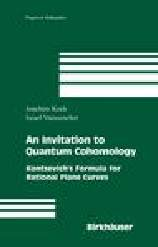Joachim's web pages [Home] [Math]## An invitation to quantum cohomology: Kontsevich's formula for rational plane curves

by Joachim Kock and Israel Vainsencher

xii+159pp., No. 249 of Progress in Mathematics, Birkhäuser, 2006.

This book is an elementary introduction to stable maps and quantum cohomology, starting with an introduction to stable pointed curves, and culminating with a proof of the associativity of the quantum product. The viewpoint is mostly that of enumerative geometry, and the red thread of the exposition is the problem of counting rational plane curves. Kontsevich's formula is initially established in the framework of classical enumerative geometry, then as a statement about reconstruction for Gromov-Witten invariants, and finally, using generating functions, as a special case of the associativity of the quantum product.

Emphasis is given throughout the exposition to examples, heuristic discussions, and simple applications of the basic tools to best convey the intuition behind the subject. The book demystifies these new quantum techniques by showing how they fit into classical algebraic geometry.

Some familiarity with basic algebraic geometry and elementary intersection theory is assumed. Each chapter concludes with some historical comments and an outline of key topics and themes as a guide for further study, followed by a collection of exercises that complement the material covered and reinforce computational skills. As such, the book is ideal for self-study, as a text for a mini-course in quantum cohomology, or as a special topics text in a standard course in intersection theory. The book will prove equally useful to graduate students in the classroom setting as to researchers in geometry and physics who wish to learn about the subject.

### Similar books

If you look for this book at flipkart.com, you will see a list of similar books, among which An Invitation to Sin by Suzanne Enoch. It has a stockinged leg on the cover. Unfortunately, from the page of that book, there is no link to An invitation to quantum cohomology...

### Errata

• ERRATUM: 1.4.3 is a bit misleading, and it is directly wrong to say "becomes singular" because the variety is already singular. The paragraph should rather read:
\begin{obs}
Observe that the stabilization of the family $\ov U_{0,4} \times_{\ov M_{0,4}}\ov U_{0,4} \to \ov U_{0,4}$ is not simply the
blowup along the intersections of the sections as it was the case
for $n=3$.  For $n\geq 4$, the projection map has singular fibres,
and it is necesssary also to blow up where the diagonal meets the
singular loci of the fibres (cf.~case-I stabilization).  In fact
this blowup results in a smooth variety.
\end{obs}